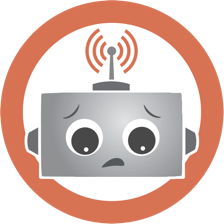The Solfeggio frequencies are inverted. And what happens when natural healing frequencies are inverted anti-clock wise? It lowers our energy, holds us back in a very low vibrational range, trapping our true natura, disconnecting us, while making us (multidimensional) sick.

The Solfeggio frequencies, worldwide famous for being Healing frequencies, or the “sounds” (vibrations) of Creation, do not match with the 3-6-9 natural order of sequence.

“If you only knew the magnificence of the 3, 6 and 9, then you would have the key to the universe.”

We are only beginning to grasp the hidden knowledge regarding the 3-6-9 mystery. The pattern starts to emerge when we add 3’s:

0 + 3 = 3

3 + 3 = 6

6 + 3 = 9

9 + 3 = 12 (1 + 2 = 3)

12 + 3 = 15 (1 + 5 = 6)

15 + 3 = 18 (1 + 8 = 9)

18 + 3 = 21 (2 + 1 = 3)

21 + 3 = 24 (2 + 4 = 6)

24 + 3 = 27 (2 + 7 = 9)

27 +3 = 30 (3); 33 (6); 36 (9); 39 (3); 42 (6) … and so on indefinitely!

The same sequence appears on the Universal numbers, referred as to “angel numbers”, triple numbers:

111 (1 + 1 + 1 = 3)

222 (2 + 2 + 2 = 6)

333 (3 + 3 + 3 = 9)

444 (4 + 4 + 4 = 12) = 3

555 (5 + 5 + 5 = 15) = 6

666 (6 + 6 + 6 = 18) = 9

777 (7 + 7 + 7 = 21) = 3

888 (8 + 8 + 8 = 24) = 6

999 (9 + 9 + 9 = 27) = 9

Can you see the pattern now?

Here are the Solfeggio Frequencies:

174 (3) – 285 (6) – 396 (9) – 417 (3) – 528 (6) – 639 (9) – 741 (3) – 852 (6) – 963 (9) Hz(Image copyrighted and trademarked by Leonard G. Horowitz)

When we separate the frequencies into the same vibrational level, we get 3 sets of triple frequencies, each with only 3 numbers:

174 (3) / 417 (3) / 741 (3)

282 (6) / 528 (6) / 852 (6)

396 (9) / 639 (9) / 963 (9)

In the above image, it shows how these 3 sets of vibrations, together form a triple tetrahedron, overlaid. If we pay close attention we notice how each edge points out to the middle number per frequency, and so it transforms into a frequency wheel:The 3 6 9 pattern, according to this “wheel”, always moves clock wise, to the right, 3-6-9, 6-9-3 and 9-3-6. It is therefore, creations natural order of manifestation and empowerment.

In comparison, the Solfeggio moves anti-clock wise, to the left, hence the 3-9-6 instead of the 3-6-9, the 6-3-9 instead of 6-9-3, and 9-6-3 instead of 9-3-6. The same with the other two sets of frequencies.

Solfeggio’s:

174 (3) – 285 (6) – 396 (9) – 417 (3) – 528 (6) – 639 (9) – 741 (3) – 852 (6) – 963 (9)

The Natural Frequencies according to the wheel:

147 (3) – 258 (6) – 369 (9) – 471 (3) – 582 (6) – 693 (9) – 714 (3) – 825 (6) – 936 (9)

These natural frequencies also can be found under the name of Solfeggio Mirror frequencies.

147 Hz:

258 Hz:

369 Hz:

471 Hz:

582 Hz:

693 Hz:

714 Hz:

825 Hz:

936 Hz:

Eli de Lemos

Some content on this page was disabled on 21 de November de 2016 as a result of a DMCA takedown notice from Leonard G. Horowitz. You can learn more about the DMCA here: